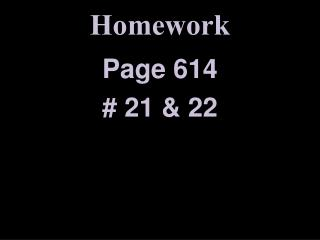DownloadDownload PresentationHomework

# Homework

Download Presentation## Homework

- - - - - - - - - - - - - - - - - - - - - - - - - - - E N D - - - - - - - - - - - - - - - - - - - - - - - - - - -
##### Presentation Transcript

1. Homework Page 614 # 21 & 22

2. The pH is given for three solutions. Calculate [H+] and [OH-] in each solution. pH = 2.37 log[H+] = -2.37 = 0.63 – 3 [H+] = 4.27 x 10-3

3. The pH is given for three solutions. Calculate [H+] and [OH-] in each solution. pH = 2.37 pOH = 14 – 2.37 = 11.63

4. The pH is given for three solutions. Calculate [H+] and [OH-] in each solution. pOH = 11.63 log[OH-] = -11.63 = 0.37 – 12 [OH-] = 2.34 x 10-12

5. The pH is given for three solutions. Calculate [H+] and [OH-] in each solution. pH = 11.05 log[H+] = -11.05 = 0.95 – 12 [H+] = 8.91 x 10-12

6. The pH is given for three solutions. Calculate [H+] and [OH-] in each solution. pH = 11.05 pOH = 14 – 11.05 = 2.95

7. The pH is given for three solutions. Calculate [H+] and [OH-] in each solution. pOH = 2.95 log[OH-] = -2.95 = 0.05 – 3 [OH-] = 1.12 x 10-3

8. The pH is given for three solutions. Calculate [H+] and [OH-] in each solution. pH = 6.50 log[H+] = -6.50 = 0.50 – 7 [H+] = 3.16 x 10-7

9. The pH is given for three solutions. Calculate [H+] and [OH-] in each solution. pH = 6.50 pOH = 14 – 6.50 = 7.50

10. The pH is given for three solutions. Calculate [H+] and [OH-] in each solution. pOH = 7.50 log[OH-] = -7.50 = 0.50 – 8 [OH-] = 3.16 x 10-8

11. Calculate the pH of the following solutions. 1.0M HI [H+] = 1.0 x 100 log[H+] = 0.00 + 0 pH = 0.00

12. Calculate the pH of the following solutions. 0.050M HNO3 [H+] = 5.0 x 10-2 log[H+] = 0.70 - 2 pH = 1.30

13. Calculate the pH of the following solutions. 1.0M KOH [OH-] = 1.0 x 100 log[OH- ] = 0.00 + 0 pOH = 0.00 pH = 14.00

14. Calculate the pH of the following solutions. 2.4 x 10-5M Mg(OH)2 [OH-] = (2.4 x 10-5) x 2 log[OH- ] = 0.68 - 5 pOH = 4.32 pH = 9.68

15. What would happen…? HCl is a strong acid. NaOH is a strong base. Both of them are dangerous… What happens if we mix them?

16. What would happen…? HCl + NaOH → ? HCl → H++ Cl- NaOH → OH- + Na+ H2O + NaCl

17. What would happen…? HCl + NaOH → H2O + NaCl acid + base → water + salt